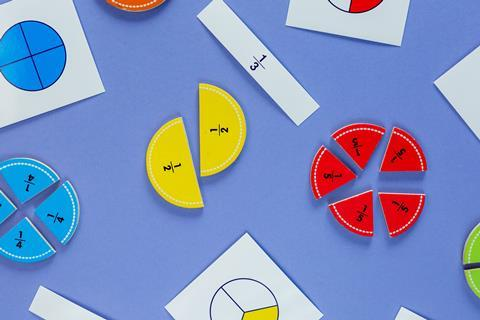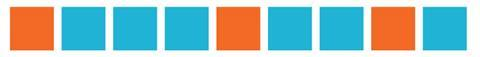Students need to be confident when handling ideas of ratio in many different chemical situations, such as analysing mixtures, working with empirical formulas and doing mole calculations. However, they often find working with ratios challenging. Even though students are taught about ratio in primary school maths lessons, it’s likely that they will retain certain misconceptions when they enter chemistry classes later in their school careers.

For example, many students will associate a ratio of 1:2 with the fraction ½ because it makes sense to use the same numbers in each case, and vice versa. Also, in trying to simplify the ratio 4:7, students may consider it to be the same as 3:6, thinking that if you subtract 1 from both the 4 and the 7, the ratio will remain the same.

There are many ways to teach ratio. Here are some ideas to try with students who need support in this area for chemistry.

#### Use familiar objects or diagrammatic representations

It’s less threatening or intimidating for students to have abstract mathematical ideas expressed diagrammatically using, for example, coloured counters or squares.#### A brief discussion

Ask students some basic questions about these orange and blue squares. Ask them how many orange squares there are, how many blue ones there are and what the total number of squares is. Then ask the students how they would compare the numbers of blue and orange squares. There are six blue squares and three orange squares – twice as many blue as orange squares.

Then introduce the word ‘ratio’. Tell students that the ratio of the number of blue to orange squares is 6:3 (as a simple comparison of number), written more simply as 2:1.

So, what does the term ‘ratio’ mean in this context? It’s being used to compare one quantity with another – the number of different coloured squares. It’s often expressed as numbers separated by a colon, or as a single number.

The ratio of blue to orange squares is 6:3 or 2:1, so there are twice as many blue as orange squares. The term ‘twice’ is used in a comparative sense here, and you could simply use the number 2, or 2:1 when referring to particular objects or things.

A worksheet introducing ratio as a concept by looking at shapes, number and some predictable chemical themes as MS Word or pdf and the answer sheet as MS Word or pdf.

A worksheet introducing ratio as a concept by looking at shapes, number and some predictable chemical themes  and the answer sheet from the Education in Chemistry website: rsc.li/xxxxxxx.

You could also say that the number of blue squares divided by the number of orange squares is 2. Or vice versa, the number of orange squares divided by blue squares is 1/2.

In this case, there are half the number of orange as blue squares, but this requires the understanding that the ‘whole’ is the ‘number of blue squares’. Instinctively, looking at the row of squares above, the ‘whole’ is ‘all of the squares’, of which the orange squares is 3/9 or 1/3.

So, there is a potential issue of what quantity is being compared to what: is it the number of coloured squares, or the total number of squares? This is why many students confuse forming a ratio with a fraction. With ratios, separate entities are being compared with each other (orange and blue squares). But when forming a fraction, an entity is being compared with a total number of entities, so of the total nine squares, three are orange, so the fraction is 1/3.

### Potential misconceptions

• When expressing a ratio, some students fail to get the part numbers in the correct order, or don’t read the parts in the correct order. The order of the ratio is given by the order of the words which describe it, and vice versa. For example, an alloy of gold and silver contains 25.0 g of gold and 10.0 g of silver, so the ratio of gold to silver is 25:10 (or 5:2), and not 10:25.
• When simplifying a ratio, some students subtract both parts rather than dividing both by a common factor. Students should explore this themselves. Teachers could draw out 10 red and five blue counters on the board or visualiser. Ask the students what the simplest ratio of red to blue counters is. Then ask whether the ratio (or proportion) of red to blue counters changes when:

1. The numbers of each colour type are multiplied by 2 or 3.

2. The numbers are divided by 5.

3. One more of each colour type is added.

4. Two more of each colour type are removed.

Students should appreciate that the value of a ratio isn’t affected by multiplying or dividing each term by the same number. However, the value of a ratio is affected by adding or subtracting the same number in each case.

When finding an equivalent ratio, some students add to both parts instead of multiplying both parts by a value. The previous activity clarifies this.

When writing a ratio as a fraction, some students place one part of the ratio over the other: 1:4 as when in fact it is 1/5. This could be remedied by using coloured shapes. For example, if 21 yellow sweets are mixed with three red sweets, the ratio of yellow to red sweets is 21:3, or 7:1. However, you could ask students what fraction of the sweets (the total number) is yellow, or red? Students should appreciate that the trigger word ‘total’ refers to the total number of sweets, so the fraction of yellow sweets is 1/(7+1) or 1/8 and red sweets is 7/(7+1) or 7/8.

Then ask why the answer is 1/8 + 7/8 = 1 when the two fractions are added together. What does the number 1 mean in this context? You could refer to slices of a cake adding up to make one whole, complete cake.

### Consider the whole picture

Some teachers may wish to launch into the chemistry problems; others may opt to start with basic ideas, building student confidence before moving on.

If students find any of these chemical calculations tricky, it may be due to a lack of understanding of the fundamental mathematics, not the chemistry (but it could also be both). Some of the calculations are challenging and more suited to those studying higher-level work, for example separate GCSE chemistry.

In these questions, the ratio work is a mere part of the solution. The ratio is set within a context, which makes it harder for students to ‘extract’ the relevant numerical information in order to form a ratio. Also, it’s very common for students to have to form a fraction, given a ratio – a common area containing misconceptions for many. The fraction is then used to calculate, for example, the mass of a component within a mixture.

Many students have different issues with maths; our students have the added complexity of using numbers within a particular chemical context. This context may be practically related, so numbers will have values with units, uncertainty and error, and a meaning or significance within their context. Also, for students to succeed in solving numerical problems in chemistry, they must be able to comprehend the literacy of the question in order to filter the appropriate relevant numerical data from the irrelevant. Possessing adequate mathematics skills is only the beginning, as students must learn to apply their maths to real chemical situations, and this high-level skill requires practice, confidence and tenacity.